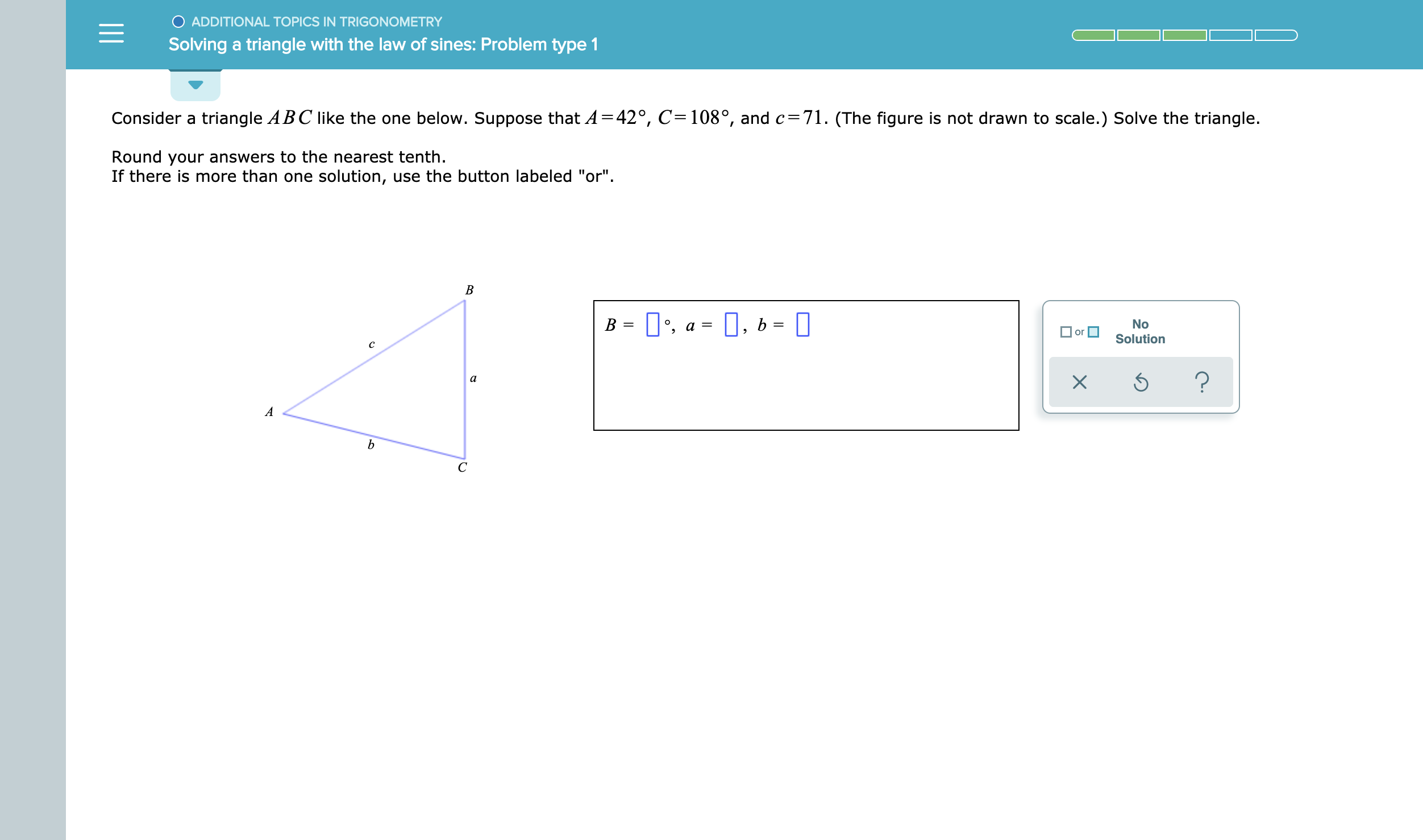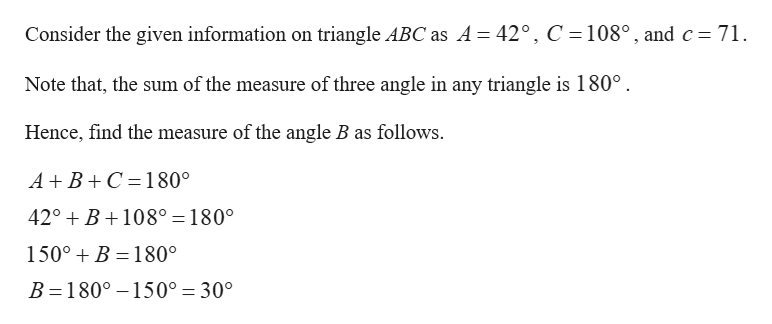O ADDITIONAL TOPICS IN TRIGONOMETRYSolving a triangle with the law of sines: Problem type 1Consider a triangle ABC like the one below. Suppose that A =42°, C=108°, and c=71. (The figure is not drawn to scale.) Solve the triangle.Round your answers to the nearest tenthIf there is more than one solution, use the button labeled "or".В, aO, bBNoor— рSolutionс?XаAb

Question

see attachmenthelp_outlineImage TranscriptioncloseO ADDITIONAL TOPICS IN TRIGONOMETRY Solving a triangle with the law of sines: Problem type 1 Consider a triangle ABC like the one below. Suppose that A =42°, C=108°, and c=71. (The figure is not drawn to scale.) Solve the triangle. Round your answers to the nearest tenth If there is more than one solution, use the button labeled "or". В , a O, b B No or — р Solution с ? X а A b fullscreen
Step 1help_outlineImage TranscriptioncloseConsider the given information on triangle ABC as A = 42°, C 108°, and c 71 Note that, the sum of the measure of three angle in any triangle is 180° Hence, find the measure of the angle B as follows. A+ B C 180° 42° B108° = 180° 150°B 180° B 180° 150° = 30° fullscreen

Want to see the full answer?

See Solution

Want to see this answer and more?

Our solutions are written by experts, many with advanced degrees, and available 24/7

See Solution
Tagged in

Other